Astronomy 162: Professor Barbara Ryden

# GENERAL RELATIVITY

``Nothing puzzles me more than time and space, and yet nothing puzzles me less, for I never think about them.'' - Charles Lamb

## Key Concept: General Relativity in One Sentence

• Mass-energy tells spacetime how to curve; the curvature of spacetime tells mass-energy how to move.

Yesterday's lecture dealt with Special Relativity, which describes how objects behave when they have a constant velocity relative to each other. Today's lecture deals with General Relativity, which describes how objects behave when they are accelerated by gravity.
Einstein's view of how gravity works is very different from Newton's view of how gravity works.

Newton's view:
Mass tells gravity how to exert a force ( F = G M1 M2 / d2 );
Force tells mass how to accelerate (F = M2 a).

Einstein's view:
Mass-energy tells spacetime how to curve;
Curvature tells mass-energy how to move.

What do we mean when we talk about the curvature of spacetime? Spacetime has four dimensions (3 space dimensions, 1 time dimension); the curvature of a four-dimensional volume is very difficult to visualize, to put it mildly!

For the purposes of visualization, consider a two-dimensional surface.

On a flat surface, like a tabletop: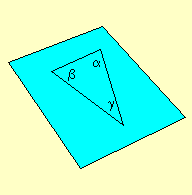The shortest distance between two points is a straight line.
Parallel lines never meet.

On a curved surface, like a globe: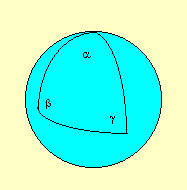The shortest distance between two points is a curved line (on a spherical globe, the shortest distance is a great circle).
Parallel lines converge or diverge (on a spherical globe, parallel lines converge).

Just as you can have flat or curved two-dimensional surfaces, you can have flat or curved four-dimensional volumes.

Curvature is amusing, but what does it have to do with gravity? Newton would say, ``Curvature is irrelevant. A massive object exerts a force on other massive objects, and makes them follow curved orbits.'' But if you asked Newton, ``What carries the force from one object to another across empty space?'', he would have to answer, ``I don't know''.

Einstein would say, ``A massive object curves the spacetime around it. Freely falling objects will follow the shortest path between two points, and hence will follow curved lines in the curved spacetime.'' Einstein would also point out that photons follow curved paths in spacetime as well, even though they have no mass.

As an analogy to Einstein's view of gravity, consider a rubber sheet which is held taut on a frame. It is flat, so if you roll a marble across it, the marble will follow a straight line. Now drop a heavy ball bearing onto the rubber sheet. The ball bearing is massive enough to cause a depression in the rubber sheet around it. Now roll a marble across the rubber sheet, close to the ball bearing. The curvature of the rubber sheet will cause the marble to follow a curved path. A distant observer, unable to see the curvature in the rubber sheet, would say ``The ball bearing must be exerting a force on the marble, deflecting it from its straight path.''

Thus, an object which is following the shortest path on a curved surface looks as if a force is being exerted on it. Similarly, an object following the shortest path in a curved four-dimensional spacetime will look as if a force (the force of gravity) is being exerted on it.

How can we tell whether Newton's view (gravity as a force) or Einstein's view (gravity as curvature) is correct? If there were no observations we could make to distinguish between the two theories, then the debate between them would be futile. However the predictions of Einstein's General Relativity theory differ from the predictions of Newton's theory in the region very close to a very massive object (where the escape speed is very close to the speed of light). Thus, General Relativity is crucial for understanding black holes, and very important for understanding neutron stars (where the escape speed from the surface is half the speed of light).

There have been three major experimental tests of the theory of General Relativity, ALL of which support Einstein over Newton.

### Test One: Bending of starlight.

Photons follow the shortest path between two points. Near a massive object, such as the Sun, the shortest path will be curved. Thus, as shown in the illustration below, the apparent position of a star close to the Sun in the sky will differ from its true position.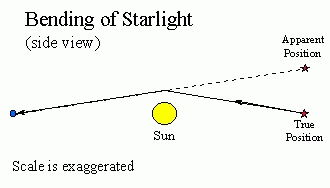The actual prediction of Einstein is that starlight passing close to the Sun will be deflected by only 1.75 arcseconds (a small but measurable amount). During a total solar eclipse in 1919, astronomers tested Einstein's prediction by photographing the apparent position of stars close to the Sun and comparing them to the true position, measured 6 months earlier when the Sun was on the opposite side of the celestial sphere. The results are shown below. The small blue circles represent the true positions of stars; the arrows point to their apparent positions when the Sun was in their midst.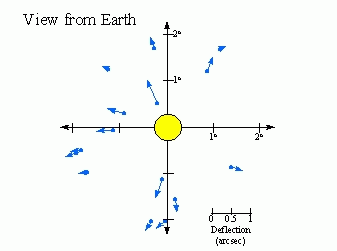The observations agree (within experimental error) with Einstein's prediction.

Score: Einstein 1, Newton 0.

As an aside: Much more spectacular examples of the bending of light are seen when light from a distant galaxy passes through a nearby galaxy or cluster of galaxies. The nearby galaxy or cluster acts as a `gravitational lens' when can cause magnified images, multiple images, and distorted images of the distant galaxy. Click on the small picture below, for instance, to see a larger image of a cluster of galaxies called Abell 2218. The extremely elongated arcs of light which you see encircling the cluster are actually badly distorted images of galaxies which lie far beyond the cluster Abell 2218.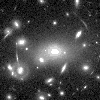[Image credit: W. Couch (U. New South Wales) & NASA]

### Test Two: Precession of the perihelion of Mercury's orbit.

The major axis of Mercury's orbit precesses - that is, it changes its position slowly with time. The effect is shown (in a greatly exaggerated form) in the illustration below: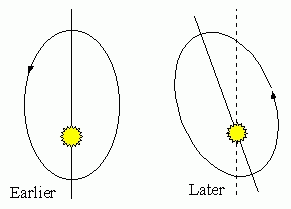Part of the precession is explained by the perturbing force of the gravity of the other planets. However, the amount of the precession is 43 arcseconds/century greater than is predicted by Newton's theory. This doesn't sound like a large effect; it will take over 3 million years to twist the orbit of Mercury through 360 degrees. However, it was large enough to throw astronomers into a tizzy at the end of the 19th century. (They actually hypothesized the presence of an additional planet, called Vulcan, with an orbit smaller than that of Mercury. )

The additional precession of 43 arcsec/century, however, is precisely equal to the precession predicted by Einstein's theory (when Mercury is at perihelion, the greater spacetime curvature gives the orbit of Mercury a little extra twist). The hunt for the planet Vulcan could be called off.

Score: Einstein 2, Newton 0.

### Test Three: Gravitational Redshift.

It takes energy to move away from a massive object. Since photons are affected by gravity, as they move away from a massive object, they must
• Decrease in energy
• Decrease in frequency
• Increase in wavelength.
Thus, light is redshifted as it moves away from a massive object. This prediction of General Relativity has been experimentally confirmed. In 1960, a pair of physicists shot a beam of gamma-rays from the bottom of a tower (23 meters tall) to a detector at the top. The redshift observed was small (only 1 part in 400 trillion!) since the Earth's gravitational field is weak, but they could detect it with the extremely sensitive detector they were using.

Score: Einstein 3, Newton 0.

It looks like Einstein wins in a shutout! Does this mean we should toss Newton in the garbage?

Not so fast...Newton's theory gives highly accurate results when speeds are slow and gravity is weak (this is true almost everywhere). Moreover, computing Newtonian forces is simpler than computing the full four-dimensional relativistic curvature, so astronomers, who like a simple life, use the theory of Newton whenever possible, resorting to the accuracy of Einstein's Theory of General Relativity only when absolutely necessary.

Prof. Barbara Ryden (ryden@astronomy.ohio-state.edu)

Updated: 2003 Feb 11